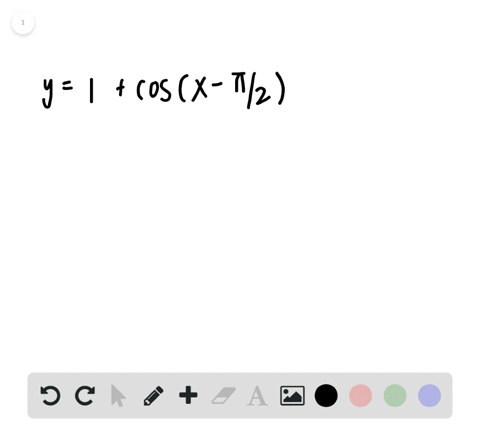🎉 The Study-to-Win Winning Ticket number has been announced! Go to your Tickets dashboard to see if you won! 🎉View Winning Ticket### Sketching the Graph of a Trigonometric Function I…

00:39University of Southern California
Problem 64

# Sketching the Graph of a Trigonometric Function In Exercises $55-66,$ sketch the graph of the function.$$y=\cos \left(x-\frac{\pi}{3}\right)$$

## Discussion

You must be signed in to discuss.

## Video Transcript

all right. Problem Number 24 asks us to create a sketch of the following function. Why equals co sign of X minus pi thirds. Now, before we begin, we should consider the general form of co sign just so we know what key values we should look for. Um, when graphing this function, it'll help us graph it later. So a, as we know, helps us find the amplitude. B is great for finding the period and see allows us to find that horizontal shift. So let's start with a well, it's simple enough. If we look here, we see that a equals one, meaning the amplitude of our function is going to be equivalent to one to find the period. Let's look for that devalue. Well, the here also equals one, meaning that the period it's gonna be that normal coastline period of two pi. Because you find a period where doing to fight about it might be if b equals one in the period is two pi. I'll just write here That amplitude equals one now see right here equals pry thirds. And because we're subtracting it, our graph is going to shift to the right, so Hi, thirds. Hi. Third shift to the right. All right, so let's draw that out. Uh huh. So let's start by grand drawing the normal coastline function that'll help us be able to see the difference created. That's right. Co sign X. No, our new function because been shifted forward. Well, look, something like this. Uh, yeah. So that Green Online is gonna be the co sign of X minus pi thirds. Just to make it pretty clear, Come in here with the black equals one that equals negative one right here to pay. I I have. And so that green graph right there is our graph of y equals Cosette of X minus pi thirds.